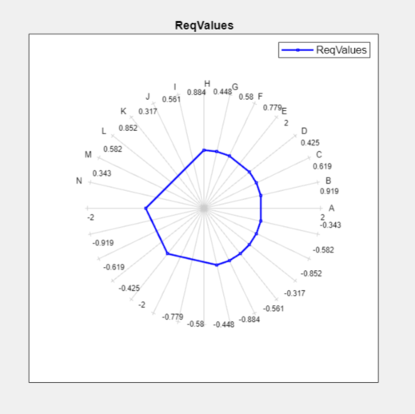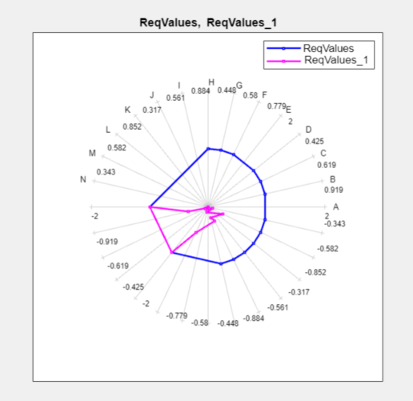## Compare Requirements and Design Variables Using Spider Plot

This example shows how to use a spider plot to compare requirement evaluations before and after optimizing the response. You can use a similar procedure to compare the values of sets of design variables.

### Open the Simulink model and load the pre-configured Response Optimization Tool session.

For this example, which uses a distillation column model, the step response requirements are preconfigured and loaded in the model workspace.

1. Open the distillation model.

```sys = 'distillation_demo'; open_system(sys)```

2. Open the Response Optimization Tool.

In the Simulink® model window, from the Apps tab, in the gallery, under Control Systems select Response Optimization.

Alternatively, click the ```Response Optimization GUI with preloaded data``` block in the model and skip the next step.

3. Load the preconfigured Response Optimization Tool session.

Click the Response Optimization tab. In the Open Session drop-down list, select Open from model workspace. A window opens where you select the Response Optimization Tool session to load. Select `distillation_optim` and click OK.

The preconfigured step response requirements are loaded in the Response Optimization Tool.

### Evaluate the requirement before optimization.

In the Response Optimization tab, click .

A new variable, `ReqValues`, containing the evaluation of the requirements appears in the Data area.

When optimizing the model response, you create a set of requirements that it must satisfy. If the requirements are violated, meaning that they evaluate to non-negative values, the design variables must be optimized. After the optimization, you can compare the original requirement value with the requirement evaluated using the optimized design variable values.

### Plot the requirement value before optimization.

1. In the Data to Plot list, select `ReqValues`.

2. In the Add Plot list, select ```Spider plot```.The plot has an axis for each edge-and-signal combination defined in the `distillation_demo/Desired Step Response` check block. Points on each axis represent the violation for that signal-edge combination and the plot connects these points to form a closed polygon representing the initial design. Note that some points are negative, representing satisfied constraints, and some positive, representing violated constraints.

### Optimize the model.

Click .

A new variable, `ReqValues1`, containing the evaluation of the requirements using the optimized design variables appears in the Data area.

### Compare the requirement values before and after optimization.

1. In the Data to Plot list, select `ReqValues1`.

2. In the Add Plot list, select ```Spider plot 1```.The optimized requirement values, `ReqValues1`, are all negative or zero, indicating that all the constraints are satisfied.

## Related Topics